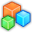# Microsoft Small BasicProgram Listing: PMT747-2
' Ruler 0.4
' Copyright (c) 2012-2013 Nonki Takahashi. All rights reserved.
'
' History :
' 0.4 2013-12-13 Fixed for remote. (PMT747-2)
' 0.3 2013-12-13 600px version. (PMT747-1)
' 0.2 2012-06-29 Ruler image version. (PMT747)
' 0.1 2012-06-29 Created.
'
GraphicsWindow.Width = 596
GraphicsWindow.Height = 426
GraphicsWindow.BackgroundColor = "White"
GraphicsWindow.BrushColor = "Green"
GraphicsWindow.Title = "Ruler 0.4"
x = 20
For i = 1 To 6
s = i * 20
GraphicsWindow.FillEllipse(x, 20, s, s)
x = x + s + 20
EndFor
Ruler_Add()
Ruler_Loop()

Sub Ruler_Add
uImage = "http://www.nonkit.com/smallbasic.files/ruler600.png"
oImage = ImageList.LoadImage(uImage)
ruler["angle"] = 0
ruler["move"] = "False"
ruler["moving"] = "False"
ruler["clicked"] = "False"
ruler["left"] = 0
ruler["top"] = 0
ruler["width"] = ImageList.GetWidthOfImage(oImage)
ruler["height"] = ImageList.GetHeightOfImage(oImage)
If ruler["width"] = 0 Then ' for remote
ruler["width"] = 620
ruler["height"] = 50
EndIf
ruler["cx"] = ruler["left"] + ruler["width"] / 2
ruler["cy"] = ruler["top"] + ruler["height"] / 2
ruler["right"] = ruler["left"] + ruler["width"]
ruler["bottom"] = ruler["top"] + ruler["height"]
ruler["oRuler"] = Shapes.AddImage(uImage)
Shapes.SetOpacity(ruler["oRuler"], 70)
Shapes.Move(ruler["oRuler"], ruler["px"], ruler["py"])
EndSub

Sub Ruler_Loop
GraphicsWindow.MouseUp = Ruler_OnMouseUp
GraphicsWindow.MouseDown = Ruler_OnMouseDown
While "True"
Program.Delay(100)
If ruler["move"] Then
ruler["move"] = "False"
x = ruler["movex"] - ruler["mousex"]
y = ruler["movey"] - ruler["mousey"]
Ruler_Move()
EndIf
If ruler["clicked"] Then
ruler["clicked"] = "False"
an = 90 - ruler["angle"]
Ruler_Rotate()
EndIf
EndWhile
EndSub

Sub Ruler_OnMouseDown
ruler["clicked"] = "N/A"
x = GraphicsWindow.MouseX
y = GraphicsWindow.MouseY
If ruler["left"] <= x And x <= ruler["right"] And ruler["top"] <= y And y <= ruler["bottom"] Then
ruler["mousex"] = x - ruler["cx"]
ruler["mousey"] = y - ruler["cy"]
ruler["move"] = "False"
GraphicsWindow.MouseMove = Ruler_OnMouseMove
ruler["moving"] = "True"
EndIf
EndSub

Sub Ruler_OnMouseUp
GraphicsWindow.MouseMove = Ruler_DoNothing
ruler["moving"] = "False"
If ruler["clicked"] = "N/A" Then
ruler["clicked"] = "True"
EndIf
EndSub

Ruler_OnMouseMove=""
Sub Ruler_OnMouseMove
GraphicsWindow.MouseMove = Ruler_DoNothing
ruler["clicked"] = "False"
ruler["move"] = "True"
ruler["movex"] = GraphicsWindow.MouseX
ruler["movey"] = GraphicsWindow.MouseY
GraphicsWindow.MouseMove = Ruler_OnMouseMove
EndSub

Sub Ruler_DoNothing
EndSub

Sub Ruler_Move
' param x, y
ruler["cx"] = x
ruler["cy"] = y
ruler["left"] = x - ruler["width"] / 2
ruler["right"] = x + ruler["width"] / 2
ruler["top"] = y - ruler["height"] / 2
ruler["bottom"] = y + ruler["height"] / 2
If ruler["angle"] = 0 Then
px = ruler["left"]
py = ruler["top"]
ElseIf ruler["angle"] = 90 Then
px = x - ruler["height"] / 2
py = y - ruler["width"] / 2
EndIf
Shapes.Move(ruler["oRuler"], px, py)
EndSub

Sub Ruler_Rotate
' param an - angle 0 or 90
If (an = 0 Or an = 90) And an <> ruler["angle"] Then
ruler["angle"] = an
Shapes.Rotate(ruler["oRuler"], an)
height = ruler["width"]
width = ruler["height"]
ruler["width"] = width
ruler["left"] = ruler["cx"] - width / 2
ruler["right"] = ruler["cx"] + width / 2
ruler["height"] = height
ruler["top"] = ruler["cy"] - height / 2
ruler["bottom"] = ruler["cy"] + height / 2
EndIf
EndSub